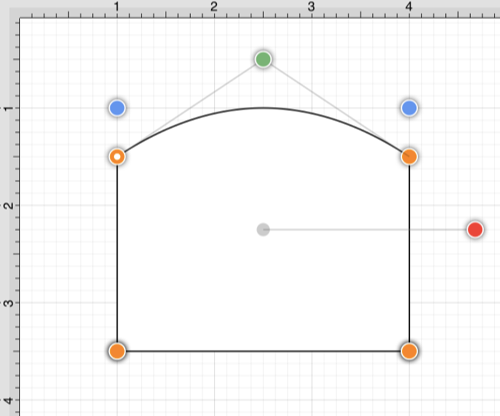The Segment to Quad action will convert either a line segment or cubic curve to a quadratic curve segment. A different behavior is received when the handle is inserted depending on the chosen segment type:

• Line Segment - A curve control point will be inserted at the center point of the line and can be used to create the exact desired quadratic.

• Cubic Curve Segment - The two control points of the cubic curve segment will be averaged to create a new control point for the quadratic curve, which is replacing the cubic curve.

In the provided example, the Line Segment located on the right side of the figure is converted into a Quadratic Curve Segment. Follow the steps below:

Step 1: Click on the desired segment to select it and then Right Click (Control-Click) to open the contextual menu.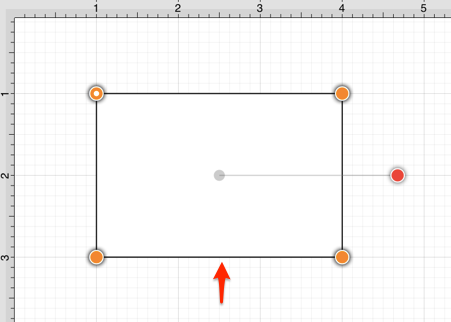Step 2: Select the Path Submenu and then choose Segment to Quad.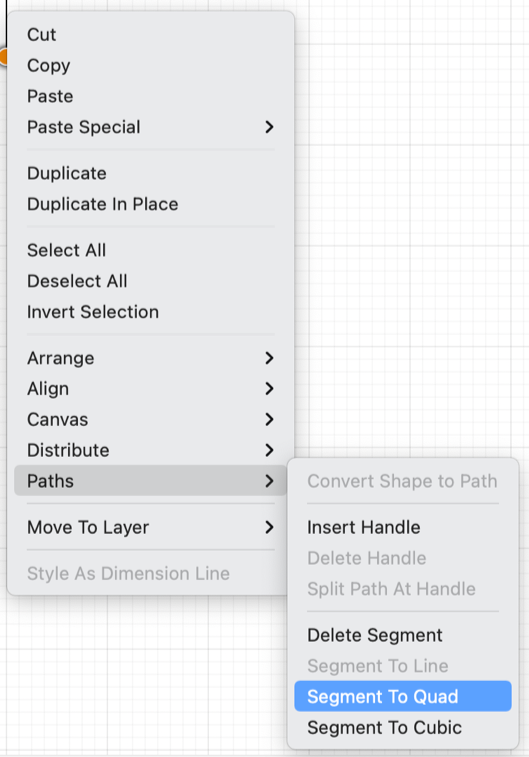Step 3: A Quadratic Curve Control Point (green) is added to the center of the selected segment.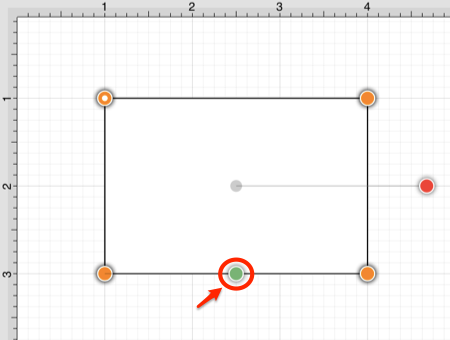Step 4: Perform a Click/Drag combination on the Quadratic Curve Control Point to create the desired curve segment. In this example, the control point is dragged in a downward direction.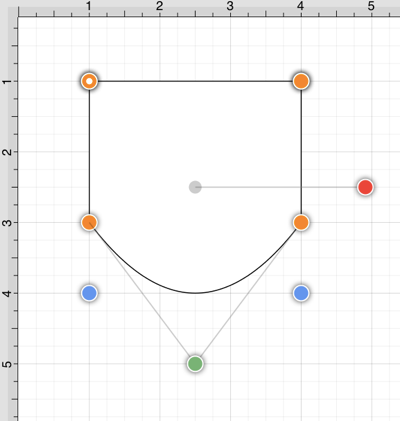The following screenshots show the resulting control point and line changes when a Cubic Curve Segment is converted to a Quadratic Curve Segment. In this example, the top cubic curve is chosen for conversion. The two existing Cubic Curve Control Points (green) are averaged to generate a single Quadratic Curve Control Point.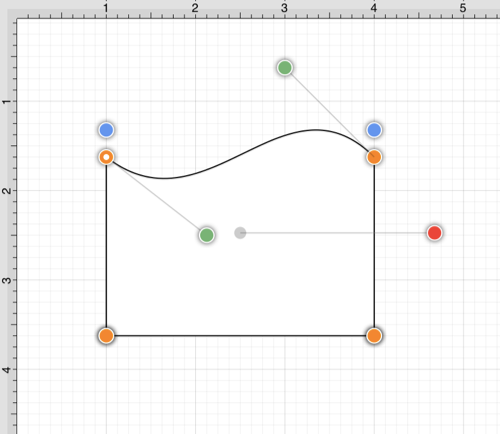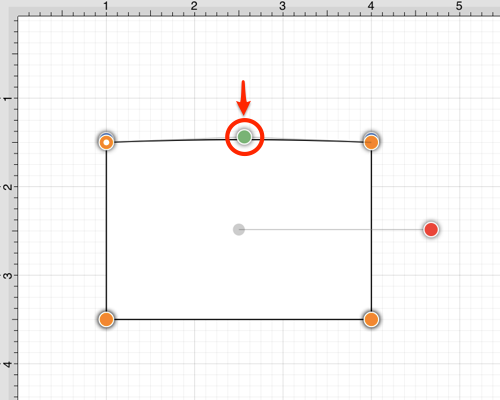The provided Quadratic Curve Control Point can be moved in any direction by performing a Click/Drag combination. In this example, the new control point is dragged upward.